# Heat Transfer Exploration of MHD Flow Stream with Changing Viscosity and Thermal Conductivity due to Expandable Surface

Heat Transfer Exploration of MHD Flow Stream with Changing Viscosity and Thermal Conductivity due to Expandable Surface

M.C. Kemparaju Bommanna Lavanya Mahantesh M. Nandeppanavar N. Raveendra

Department of Mathematics, Jyothi Institute of Technology (CIIRC), Bangalore 560065, India

Department of Mathematics, Manipal Institute of Technology, Manipal Academy of Higher Education, Manipal 576104, Karnataka, India

Department of Mathematics, Government College (Autonomous), Kalaburagi 585101, India

Department of Mathematics, Rajarajeswari College of Engineering, Bangalore 560074, India

Corresponding Author Email:
lavanya.b@manipal.edu
Page:
955-960
|
DOI:
https://doi.org/10.18280/mmep.080615
14 September 2021
|
Accepted:
18 November 2021
|
Published:
22 December 2021
| Citation

OPEN ACCESS

Abstract:

In this paper an examination is completed to explore the influence of variable thickness and variable thermal conductivity on MHD stream. We have considered the governing stream and heat transfer conditions as partial differential equations. These non-linear partial differential equations are changed to non-linear ordinary differential equations at that point explained numerically utilizing fourth order RK strategy with shooting procedure. The influence of governing factors on velocity and temperature is concentrated through diagrams and numerical estimations of skin frictions and wall temperature inclination are determined, classified and examined.

Keywords:

MHD, variable viscosity, variable thermal conductivity, stretching sheet

1. Introduction

Iinvestigation of viscous incompressible fluid stream over an extending sheet and heat transfer has an extraordinary enthusiasm due to its industrial applications. the extraction of plastic sheets, sheet metals, polymer extraction, wire drawing, glass blowing, casting of metals etc. are practical applications, the cooling process and heat transfer play vital role in manufacturing of these products. Accordingly, Sakiads  examine the borderline layer performance on the continuous solid surface. Later on, Gupta, P.S. and Gupta, A.S.  investigated the impact of heat and mass transmission over a widening surface with the forces of pull or blowing. Chakarbarti and Gupta  inspect hydro magnetic stream heat transfer on extending sheet. Grubka and Bobba  broke down the impact of heat transmission attributes of a continuous extending surface with inconstant temperature.

2. Mathematical Formulation

Here steady two dimensional limit layer stream of an incompressible fluid streams past an extending sheet is thought of, equivalent and inverse powers acting along the x-axis and uniform attractive field B0 is applied along y-axis normally. The variable thickness and variable warm conductivity are fluctuating and different boundaries are to be expected as constant. The governing equations are given by:

$\frac{\partial u}{\partial x}+\frac{\partial v}{\partial y}=0$        (1)

$u\frac{\partial u}{\partial x}+v\frac{\partial u}{\partial y}=\frac{1}{\rho }\frac{\partial }{\partial y}\left( \mu \frac{\partial u}{\partial y} \right)-\frac{\sigma {{B}_{0}}^{2}}{\rho }u$                (2)

$\rho {{c}_{p}}\left( u\frac{\partial T}{\partial x}+v\frac{\partial T}{\partial y} \right)=\frac{\partial }{\partial y}\left( k\frac{\partial T}{\partial y} \right)$          (3)

The boundary restrictions are:

\begin{align} & u=Bx,\,\,\,\,\,v=0,\,\,\,\,\,T={{T}_{w}}\,\,\,\,\,at\,\,y=0 \\ & u\to 0,\,\,\,\,\,\,T\to {{T}_{\infty }}\,\,\,\,\,as\,\,\,\,\,y\to \infty \\ \end{align}           (4)

By introducing similarity transformation and dimensionless quantities as:

$\eta =\sqrt{\frac{B}{\upsilon }}y,\,\,\,u=BxF'(\eta ),\,\,\,\,\,\,v=-\sqrt{B\upsilon }F(\eta ), \\ \theta =\frac{T-{{T}_{\infty }}}{{{T}_{w}}-{{T}_{\infty }}}$         (5)

The temperature reliant on fluid viscosity is given by:

$\mu ={{\mu }^{*}}(a+b({{T}_{w}}-T))$        (6)

$\mu=\frac{1}{\left(b_{1}+b_{2} T\right)}$ is viscosity- temperature and can be expressed in the form:

$\mu=\frac{1}{b_{1}}\left(1+\frac{b_{2}}{b_{1}} T\right)^{-1}=\frac{1}{b_{1}}\left(1-\frac{b_{2}}{b_{1}} T+\frac{b_{2}^{2}}{b_{1}^{2}} T^{2}-\cdots \ldots . .\right) \cong \frac{1}{b_{1}}-\frac{b_{2}}{b_{1}} T$

$\mu=a-b T$

where, $a=\frac{1}{b_{1}}, b=\frac{b_{2}}{b_{1}}$.

The value of $b_{1}=53.41, b_{2}=2.43$ are to be taken and it gives $0^{0} \leq T \leq 23^{0}$. The viscosity-temperature relation satisfies the relation $\mu=e^{-a T}$. In this expression, the 2nd and further order terms are ignored and the vary of temperature $\left(T_{w}-T_{\infty}\right)$ is considered i.e. $\left(0^{0}-23^{0}\right)$. We expect that thermal conductivity of the fluid is a linear function of the temperature and can be written as:

$k={{k}_{\infty }}\left( 1+\varepsilon \frac{T-{{T}_{\infty }}}{{{T}_{w}}-{{T}_{\infty }}} \right),\,\,\,\,\,\,k={{k}_{\infty }}\left( 1+\varepsilon \theta (\eta ) \right)$             (7)

The governing Eqns. (2)-(4) are converted by using Eq. (4)-(7) and can be transcribed as:

\begin{align} & \left[ a+A(1-\theta ) \right]F'''-AF''\theta ' \\ & +FF''-F{{'}^{2}}-MF'=0 \\ \end{align}        (8)

$\left( 1+\varepsilon \theta \right)\theta ''+\Pr F\theta '=0$        (9)

Here $A=b\left(T_{w}-T_{\infty}\right)$ is variable viscosity, $M=\frac{\sigma B_{0}^{2}}{\rho \beta}$ and $\operatorname{Pr}=\frac{\mu c_{p}}{\kappa_{\infty}}$.

The boundary conditions (4) can be reduced:

$F^{\prime}=1, F=0, \theta=1$ at $\eta=0$

$F^{\prime} \rightarrow 0, \theta \rightarrow 0$ as $\eta \rightarrow \infty$               (10)

The physical quantities of friction coefficent $C_{f}$ and the local Nusselt number $N u_{x}$ which are defined as:

${{C}_{f}}=\frac{{{\tau }_{w}}}{\rho u_{e}^{2}(x)},\,\,\,\,\,\,\,N{{u}_{x}}=\frac{x{{q}_{w}}}{k({{T}_{w}}-{{T}_{\infty }})}$            (11)

Here $\tau_{w}$ and $q_{w}$ are sheared stress and heat flux at the wall is given by:

${{\tau }_{w}}=\mu {{\left( \frac{\partial u}{\partial y} \right)}_{y=0}},\,{{q}_{w}}=-k{{\left( \frac{\partial T}{\partial y} \right)}_{y=0}}$           (12)

Substituting (5) into (11) and (12):

$\operatorname{Re}_{x}^{\frac{1}{2}}{{C}_{f}}=F''(0),\,\,\,\,\,\,\operatorname{Re}_{x}^{-\frac{1}{2}}N{{u}_{x}}=\theta '(0)\,\,$             (13)

Here $R e_{x}=\frac{B}{v} x^{2}$ is local Reynolds number.

3. Numerical Solution

\left. \begin{align} & {{x}_{1}}=F,{{x}_{2}}=F',{{x}_{3}}=F'',{{x}_{4}}=\theta ,{{x}_{5}}=\theta ' \\ & {{x}_{1}}'={{x}_{2}},\,\, \\ & {{x}_{2}}'={{x}_{3}},\, \\ & {{x}_{3}}'=\,\frac{1}{(a+A(1-{{x}_{4}}))} \\ & (A{{x}_{3}}{{x}_{5}}-{{x}_{1}}{{x}_{3}}+x_{2}^{2}+M{{x}_{2}}), \\ & {{x}_{4}}'={{x}_{5}} \\ & {{x}_{5}}'=-\Pr {{x}_{1}}{{x}_{5}} \\ \end{align} \right\}                        (14)

The administering halfway differential conditions are hard to settle, so they are changed over into conventional differential conditions as Eqns. (8) and (9) by using similarity transformations. These BVP’s Eqns. (8) and (9) are converted into first order ODEs (i.e. into IVP’s), as shown in Eq. (14).

The corresponding boundary conditions (11) are transmuted in to:

\left. \begin{align} & {{x}_{1}}(0)=0,\,\,\,\,\,\,\,\,\,{{x}_{2}}(0)=1,\,\,\,\,\,\,\,{{x}_{4}}(0)=1 \\ & {{x}_{2}}(\infty )=0,\,\,\,\,\,\,\,\,\,\,\,\,\,\,{{x}_{4}}(\infty )=0 \\ \end{align} \right\}                 (15)

We select the appropriate estimate values to F''(0) and θ'(0) which satisfy the identical conditions F'(∞) and θ(∞) using shooting method constantly until the desired values are attained.

4. Results and Discussion

The outcomes acquired from the arrangement of framework Eqns. (8) and (9) by utilizing Runge-Kutta strategy is utilized to comprehend coupled ordinary differential equations with limit conditions Eq. (10) for various estimations of different boundary. The numerical estimations of wall temperature slope -θ'(0) are tabulated in Table 1. The similar investigation of our outcomes with prior outcomes.

Table 1. Standards of wall temperature gradient $-\theta^{\prime}(0)$ for distinct estimations of Pr with a=1, A=0, b=0 and M=0

 Pr a Gupta and Gupta  Grubka and Bobba  Ali  Eldahab and Aziz  Abel  Present Results 0.5 1 0.4631 0.45255 0.45445 0.4631 0.463144 1 1 0.582 0.582 0.59988 0.58201 0.58197 0.581976 10 1 2.308 2.29589 2.30801 2.308 2.308004

The current outcomes are awesome concurrence with existing outcomes. Table 2 exhibits the estimations of friction coefficient -F''(0) and the wall temperature gradient -θ'(0) for various standards of viscosity factor A and thermal conductivity parameter ε with a=1 and Pr=0.71, M=0.5. In this table in the event that expanding of thickness boundary, at that point both friction coefficient and wall temperature, inclination are improvements and expanding of thermal conductivity boundary, the wall temperature gradient -θ'(0) diminishes.

Table 3 presents the estimations of friction factor -F''(0) and the wall temperature, inclination -θ'(0) for various estimations of Prandtl group Pr, Magnetic factor M and thermal conductivity factor ε with a=1 and A=1. In this table expanding of Prandtl number, then both friction coefficient and wall temperature gradient are improvements. Expanding of Magnetic boundary, the skin contact coefficient increments and wall temperature inclination diminish. Both friction coefficient and the wall temperature, angles are diminished by expanding of thermal conductivity.

Table 2. Friction coefficient -F''(0), wall temperature gradient -θ'(0) standards with a=1 and Pr=0.71, M=0.5

 A ε -F''(0) -θ'(0) 0 0 1.224745 0.416435 1 0 1.307836 0.429777 4 0 1.548885 0.452397 5 0 1.625417 0.457112 6 0 1.700122 0.461081 0 0.01 1.224745 0.415144 1 0.01 1.307631 0.428486 4 0.01 1.548198 0.451126 5 0.01 1.624597 0.455848 6 0.01 1.699179 0.459823 0 0.1 1.224745 0.404028 1 0.1 1.305853 0.417356 4 0.1 1.54221 0.440176 5 0.1 1.617456 0.444954 6 0.1 1.690965 0.448978 0 0.2 1.224745 0.392631 1 0.2 1.303996 0.40593 4 0.2 1.535946 0.428923 5 0.2 1.609981 0.433758 6 0.2 1.682366 0.437833

Table 3. Friction coefficient -F''(0) and the wall temperature gradient -θ'(0) standards with a=1 and A=1

 Pr M ε -f''(0) -θ'(0) 0.5 0.5 0.01 1.289633 0.326837 0.71 0.5 0.01 1.307631 0.428486 2 0.5 0.01 1.374268 0.873445 7 0.5 0.01 1.468916 1.845448 10 0.5 0.01 1.495678 2.254399 100 0.5 0.01 1.632231 7.687693 7 1 0.01 1.679278 1.804895 7 2 0.01 2.021271 1.73795 7 3 0.01 2.301542 1.682451 7 5 0.01 2.760284 1.59121 7 0.5 0.1 1.466952 1.811691 7 0.5 0.2 1.464865 1.776944 7 0.5 0.3 1.46287 1.744711 7 0.5 0.4 1.460957 1.714661 7 0.5 0.5 1.459119 1.686527

Expanding of thermal conductivity, then Figure 1 outlines the impacts of temperature reliant on variable viscosity factor A in the velocity profile. The graph illustrates that the velocity sketch increments with expanding movable viscosity parameter. The impacts of a magnetic factor if there should be an occurrence both unchanging viscosity and adjustable viscosity, the velocity profile diminishes with expanding of magnetic parameter, it appears in Figure 2 and Figure 3.

The impact of Prandtl number Pr in the event of adjustable viscosity on velocity study is appeared in Figure 4.

The impacts are improved when the Prandtl number is increments. Figure 5 displays that the influence of variable viscosity A on temperature profile impacts is reduced by extending adjustable viscosity. Figure 6 exhibits that the temperature profile improves with extending thermal conductivity limit ε if there ought to emerge an event of adjustable viscosity.

The impacts of magnetic boundary M in both uniform viscosity and variable viscosity, the temperature profile increments with expanding attractive boundary and it tends to be appeared in Figure 7 and Figure 8. Figure 9 and Figure 10 display that the temperature profile diminishes if there should arise an occurrence of both uniform viscosity and variable viscosity when Prandtl number Pr increments.

## 1.png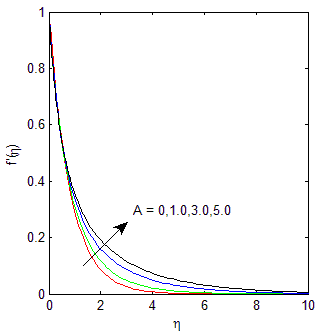Figure 1. Velocity profile for distinct estimations of variable viscosity A when a=1, Pr=0.71, M=0.5 & ε=0.5

## 2.pngFigure 2. Velocity profile for various estimations of Magnetic Factor M in the case of uniform viscosity when a=1, Pr=0.71, ε=0.5 & A=0

## 3.pngFigure 3. Velocity profile for distinct estimations of Magnetic factor M In the case of variable viscosity when a=1, Pr=0.71, ε=0.5 & A=1

## 4.png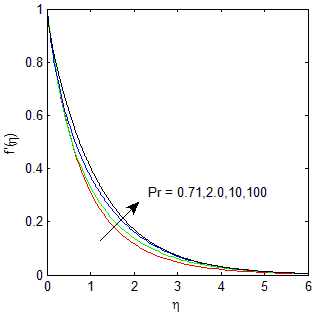Figure 4. Velocity description for different estimations of Magnetic factor M in case of variable viscosity when a=1, M=0.5, ε=0.5 & A=0

## 5.png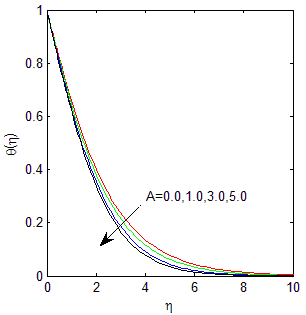Figure 5. Temperature profile for distinct standards of variable viscosity when a=1, Pr=0.71, M=0.5 & ε=0.5

## 6.png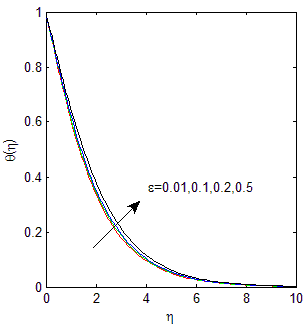Figure 6. Temperature sketches for various estimations of movable thermal Conductivity ε in the case of variable viscosity when a=1, Pr=0.71, M=0.5 & A=1

## 7.png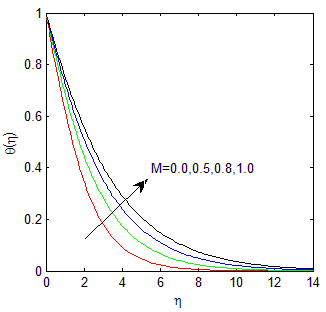Figure 7. Temperature descriptions for many estimations of a magnetic factor M In the case of uniform viscosity when a=1, Pr=0.71, ε=0.5 & A=0

## 8.png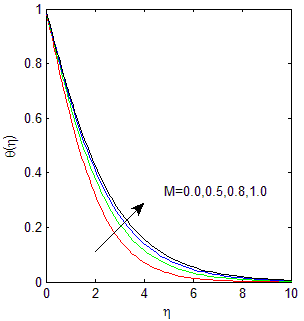Figure 8. Temperature sketches for distinct estimations of a magnetic factor M In the case of variable viscosity when a=1, Pr=0.71, ε=0.5 & A=1

## 9.png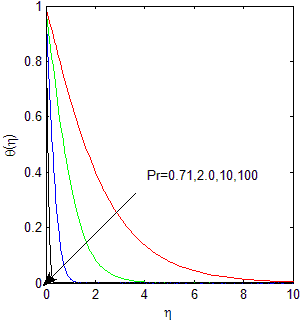Figure 9. Temperature sketches for many estimations of a magnetic factor M In the case of uniform viscosity when a=1, M=0.5, ε=0.5 & A=0

## 10.png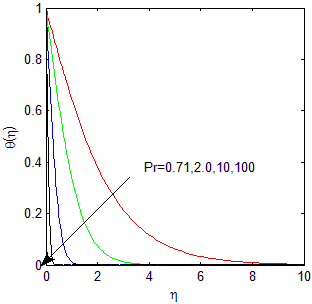Figure 10. Temperature sketches for many estimations of a magnetic factor M In the case of variable viscosity when a=1, M=0.5, ε=0.5 & A=0

5. Conclusion

The two dimensional MHD stream and heat move over an extending sheet has been contemplated. The impacts of different physical boundaries like Prandtl number Pr, variable viscosity A, Magnetic Parameter M and variable warm conductivity ε are examined through tables and graphs. The outcome of the study shows that the stream velocity and skin friction co-efficient -F''(0) on extending sheet are strongly influenced by magnetic parameter M and variable viscosity A. Similarly the heat transfer and wall temperature gradient -θ'(0) are influenced by variable thermal conductivity. From the present paper we investigate the following brief conclusion may be drawn.

1. An increment in magnetic parameter M and variable viscosity A, the skin friction coefficient diminishes.
2. Expanding in Prandtl group Pr and adjustable thermal conductivity ε, the skin friction coefficient diminishes.
3. An increment in thermal conductivity ε, wall temperature gradient will improve.

The stream conduct is comparative, if expanding in attractive boundary in nearness of both uniform viscosity and variable viscosity.

Acknowledgments

Authors are thankful to the referee for the valuable suggestions who help to improve the quality of this manuscript and also support of our institutional departments.

References

 Sakiadis, B.C. (1961). Boundary layer behavior on continuous solid surface. AIChE Journal, 7(2): 221-225. https://doi.org/10.1002/aic.690070108

 Gupta, P.S., Gupta, A.S. (1977). Heat and mass transfer on a stretching sheet with suction or blowing. The Canadian journal of Chemical Engineering, 55(6): 744-746. https://doi.org/10.1002/cjce.5450550619

 Chakrabarti, A., Gupta, A.S. (1979). Hydromagnetic flow and heat transfer over a stretching sheet. Quarterly of Applied Mathematics, 37(1): 73-78. https://www.jstor.org/stable/43636961

 Grubka, L.J., Bobba, K.M. (1985). Heat transfer characteristics of a continuous, stretching surface with variable temperature. Journal of Heat Transfer, 107: 248-250. https://doi.org/10.1115/1.3247387

 Vajravelu, K., Rollins, D. (1992). Heat transfer in an electrically conducting fluid over a stretching surface. International Journal of Non-linear mechanics, 27(2): 265-277. https://doi.org/10.1016/0020-7462(92)90085-L

 Char, M.I. (1994). Heat transfer in a hydromagnetic flow over a stretching sheet. Wärme-und Stoffübertragung, 29(8): 495-500. https://doi.org/10.1007/BF01539502

 Ali, M.E. (1995). On thermal boundary layer on a power-law stretched surface with suction or injection. International Journal of Heat and Fluid Flow, 16(4): 280-290. https://doi.org/10.1016/0142-727X(95)00001-7

 Andersson, H.I. (2002). Slip flow past a stretching surface. Acta Mechanica, 158(1): 121-125. https://doi.org/10.1007/BF01463174

 Abo-Eldahab, E.M., El Aziz, M.A. (2004). Blowing/suction effect on hydromagnetic heat transfer by mixed convection from an inclined continuously stretching surface with internal heat generation/absorption. International Journal of Thermal Sciences, 43(7): 709-719. https://doi.org/10.1016/j.ijthermalsci.2004.01.005

 Cortell, R. (2006). Flow and heat transfer of an electrically conducting fluid of second grade over a stretching sheet subject to suction and to a transverse magnetic field. International Journal of Heat and Mass Transfer, 49(11-12): 1851-1856. https://doi.org/10.1016/j.ijheatmasstransfer.2005.11.013

 Mahmoud, M.A. (2007). Thermal radiation effects on MHD flow of a micropolar fluid over a stretching surface with variable thermal conductivity. Physica A: Statistical Mechanics and Its Applications, 375(2): 401-410. https://doi.org/10.1016/j.physa.2006.09.010

 Abel, M.S., Mahesha, N. (2008). Heat transfer in MHD viscoelastic fluid flow over a stretching sheet with variable thermal conductivity, non-uniform heat source and radiation. Applied Mathematical Modelling, 32(10): 1965-1983. https://doi.org/10.1016/j.apm.2007.06.038

 Abdou, M.M.M. (2010). Effect of radiation with temperature dependent viscosity and thermal conductivity on unsteady a stretching sheet through porous media. Nonlinear Analysis: Modelling and Control, 15(3): 257-270. https://doi.org/10.15388/NA.15.3.14322

 Mahmoud, M.A. (2010). Flow and heat transfer of a slightly rarefied gas over a stretching surface. Meccanica, 45(6): 911-916. https://doi.org/10.1063/1.4967599

 Kandasamy, R., Muhaimin, I., Saim, H.B. (2010). Lie group analysis for the effect of temperature-dependent fluid viscosity with thermophoresis and chemical reaction on MHD free convective heat and mass transfer over a porous stretching surface in the presence of heat source/sink. Communications in Nonlinear Science and Numerical Simulation, 15(8): 2109-2123. https://doi.org/10.1016/j.cnsns.2009.09.016

 Pal, D. (2011). Combined effects of non-uniform heat source/sink and thermal radiation on heat transfer over an unsteady stretching permeable surface. Communications in Nonlinear Science and Numerical Simulation, 16(4): 1890-1904. https://doi.org/10.1016/j.cnsns.2010.08.023

 Mukhopadhyay, S., Layek, G.C. (2012). Effects of variable fluid viscosity on flow past a heated stretching sheet embedded in a porous medium in presence of heat source/sink. Meccanica, 47(4): 863-876. https://doi.org/10.1007/s11012-011-9457-6

 Dessie, H., Kishan, N. (2014). MHD effects on heat transfer over stretching sheet embedded in porous medium with variable viscosity, viscous dissipation and heat source/sink. Ain Shams Engineering Journal, 5(3): 967-977. https://doi.org/10.1016/j.asej.2014.03.008

 Daniel, Y.S., Daniel, S.K. (2015). Effects of buoyancy and thermal radiation on MHD flow over a stretching porous sheet using homotopy analysis method. Alexandria Engineering Journal, 54(3): 705-712. https://doi.org/10.1016/j.aej.2015.03.029

 Tripathy, R.S., Dash, G.C., Mishra, S.R., Baag, S. (2015). Chemical reaction effect on MHD free convective surface over a moving vertical plate through porous medium. Alexandria Engineering Journal, 54(3): 673-679. https://doi.org/10.1016/j.aej.2015.04.012

 Bhukta, D., Dash, G.C., Mishra, S.R., Baag, S. (2017). Dissipation effect on MHD mixed convection flow over a stretching sheet through porous medium with non-uniform heat source/sink. Ain Shams Engineering Journal, 8(3): 353-361. http://dx.doi.org/10.1016/j.asej.2015.08.017

 Bayones, F.S., Nisar, K.S., Khan, K.A., Raza, N., Hussien, N.S., Osman, M.S., Abualnaja, K.M. (2021). Magneto-hydrodynamics (MHD) flow analysis with mixed convection moves through a stretching surface. AIP Advances, 11(4): 045001. https://doi.org/10.1063/5.0047213

 Reddy, N.N., Rao, V.S., Reddy, B.R. (2021). Chemical reaction impact on MHD natural convection flow through porous medium past an exponentially stretching sheet in presence of heat source/sink and viscous dissipation. Case Studies in Thermal Engineering, 25: 100879. https://doi.org/10.1016/j.csite.2021.100879